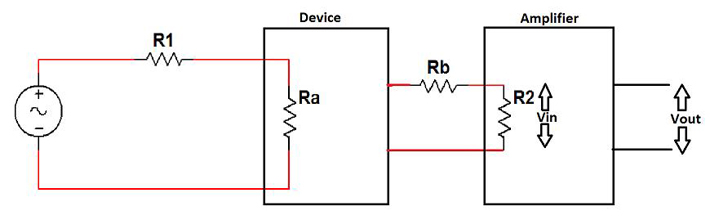﻿ Impedance Matching & Buffers | EngineersGarage
Close or Esc Key

Impedance Matching & Buffers

Written By:

Ajit Matthew
Sometimes we design and make circuits which function correctly in pieces but when the circuits are connected together, they do not give the expected result. For example, design a low frequency oscillator such as a Wien bridge oscillator and a common collector amplifier. Check their functioning separately and then connect the output of the oscillator to the input of the amplifier. If the design of the amplifier is not meticulous, most probably the output will not be as expected. This may be due to ignorance of a simple but a very important concept called impedance matching.
So what is impedance matching?  To understand this let us take a classic example where impedance matching is used, sound amplification using a microphone and a loudspeaker.   Microphone is a device which converts sound energy into electrical energy and hence can be modelled as a signal generator with source resistance, typical value of source resistance is around 500 ohms (R1). A loudspeaker is basically a signal amplifier in addition to a device which can convert electrical energy into sound energy. Typically, a loudspeaker has input resistance of about 10 ohms(R2).  The whole circuit can be modelled as shown in the figure.Fig. 1: Equivalent Circuit Diagram of a Loudspeaker

If the output of the microphone is 50mV and k=100, then Vin will approximately equal to 1mV(R2*50/(R1+R2)) and hence Vout=100mV, which will not be sufficient to drive the speaker. Here, R2 is much smaller than R1 and hence most of the voltage produced by the microphone shows up at the source resistance not at the amplifier. This situation is an impedance mismatch or “loading”. Ideally, we want the whole of the input voltage to appear across R2. So how to increase the output voltage? A simple solution can be to increase amplification factor K of the amplifier. But this is not a very good idea because when we amplify very small signal we also amplify the omnipresent and irremovable noise. So the output signal will be highly distorted due to noise.  Also to increase amplification we may have to put amplifiers in cascade which will increase the cost of the circuit.
Another solution is to increase R2 or reduce R1, so that the voltage drop across R2 will increase and hence Vout will increase. But R1 and R2 cannot be changed much as they are present due to the design of the microphone and the loudspeaker.This brings us to the concept of BUFFERS.
BUFFERS
Imagine a device that has an input resistance much greater that the source resistance of the microphone say 50K ohm (Ra) and output resistance much less than input resistance of amplifier say 1 ohm (Rb). Also let the design of the device be such that input voltage is almost equal to output voltage that is    Vout/Vin=1. If we use this device as an intermediate stage between the microphone and the amplifier we might be able to solve the problem. Let us see how?Fig. 2: Equivalent Circuit Diagram of Buffers

Voltage across Ra=49.5 mV (Using voltage divider rule)
Voltage across Rb+R2=Voltage across Ra=49.5mV (According to the design of the device, Vout/Vin=1)
Voltage across R2= 45 mV (Using voltage divider rule)
Vout= 45 x 100 = 4.5V
We can see that the output voltage has increased 10 times and now the voltage is sufficient to drive the speaker. Also we will see further thatbuffer provide current gain which is crucial to drive the output. Hence this device solves our problem.
Buffer Design
So, is it possible to design such a device with a high input resistance, low output resistance and gain almost equal to one. Well, the answer is yes, it is quite possible.
Using Transistor:
A common collector amplifier acts as buffer. As for a biased transistor, Vbe=0.7V, so Vout=Vin -0.7V and hence the gain of the amplifier is almost 1.Fig. 3: Circuit Diagram of Transistor as Buffer

The approximate value of input resistance of this circuit is ?Re where ? is the current gain of the transistor. The output resistance is Rs/?where Rs is the resistance of the source driving the base of transistor. Typical values of ? is around 50 to 100, and hence the input resistance of this circuit is high and output resistance is low. A more practical circuit is as follows:Fig. 4: Circuit Diagram of Transistor as Buffer

Transistor with higher value of ?should be chosen to improve the input and output resistance.

OPAMP BUFFERS

OPAMP BUFFERS
Opamps have high input resistance (around 1Mohms) and low output resistance (typically tens of ohms) and hence can be used to make a buffer. Unity gain is achieved by applying a full negative feedback in series.Fig. 5: Circuit Diagram of OPAMP Buffer
In case subsequent gain stages invert the signal, one can use the following buffer which gives an inverted signal and hence the final output will be in phase with the initial signal.Fig. 6: Circuit Diagram of OPAMP Buffer
Current Buffer
Buffers discussed till now are used to transfer voltage and hence are called “Voltage buffers” or “Voltage follower”. But there is another kind of buffer called “Current buffer” which transfer current from one circuit to another but are not widely used as mostly we deal with voltage amplifiers. Current buffers have low input resistance and high output resistance and their current gain is unity. A common base amplifier can be used as a current buffer.